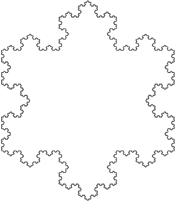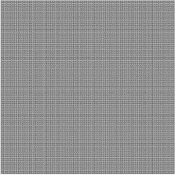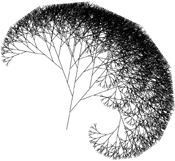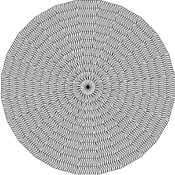## Generating Fractals - Examples

In the examples below, clicking on the image will open a PostScript slide show of the consecutive iterations of that particular fractal. The PostScript files are huge, so they have been placed in ZIP files. When you click on an image, choose to save the ZIP file to disk. Once it has completed, choose to open the file, and then double-click the PostScript file contained in the ZIP file to run the slide show

Note that if the first iteration is blank, it is not part of the slide show The first iteration is blank if the initial axiom contains no movement characters; that is, if the turtle doesn't move during translation of the axiom into an image.

### Koch SnowflakeInitial Axiom F++F++F Transformation Rules F > F-F++F-F Initial Heading (degrees) 0 Rotation Unit (degrees) 60

One of the best-known Lindenmayer fractals, the Koch snowflake is produced using quite a simple Lindenmayer grammar, as fractals go. It is named after Helge von Koch, who described it in 1904. Also known as the Koch curve. It can also be called the Koch island, but this can be confused with the quadratic Koch island below.Initial Axiom F Transformation Rules G > F+G+F F > G-F-G Initial Heading (degrees) 0 Rotation Unit (degrees) 60

The Sierpinski gasket is another well-known Lindenmayer fractal. It was first formally described by Sierpinski in 1915 although the idea had appeared in artwork for centuries. It has also been called the Sierpinski sieve or the Sierpinski triangle.

There is more than one Lindenmayer grammar which will build this fractal. The one used here illustrates the purpose of having two different letters for movement, F and G. When the turtle translates the current axiom into an image, it treats an F or G as exactly the same thing -- a line straight ahead. But when the transformation rules are applied to produce the next axiom, the F's and G's are treated separately and are replaced with different strings of characters.

Conceptually the gasket can be produced by starting with a filled equilateral triangle and removing the inner triangle which is formed by placing its vertices at the midpoints of the original triangle's edges. (This part being removed is the largest white triangle in the image on the left.) This produces three smaller filled equilateral triangles. At each step, remove the inner triangle from every filled triangle. As this process is repeated indefinitely, the Sierpinski gasket is formed.

### Hilbert CurveInitial Axiom X Transformation Rules X > -YF+XFX+FY- Y > +XF-YFY-FX+ Initial Heading (degrees) 0 Rotation Unit (degrees) -90

Invented by German mathematician David Hilbert in 1891, the Hilbert curve is an example of what is called a plane-filling curve. A plane-filling curve is a line which completely fills a two-dimensional area. The image on the left shows that after only 8 iterations, the square is already almost completely covered. As the number of iterations approaches infinity, the resulting fractal crosses every point in the plane.Initial Axiom F+F+F+F Transformation Rules F > F+F-F-FF+F+F-F Initial Heading (degrees) 45 Rotation Unit (degrees) -90

The quadratic Koch island can simply be called the Koch island. This is a modification of the Koch snowflake, mentioned by Mandelbrot in 1982. Mandelbrot is responsible for a huge amount of work and research in the field of fractals, and in fact has an entire field of fractal types named after him.

### WillowInitial Axiom LFFF Transformation Rules LFFF > TFFF[+LFFF]RFFF RFFF > TFFF[-LFFF]LFFF TF > TFF Initial Heading (degrees) 80 Rotation Unit (degrees) -30

This fractal is less known than those mentioned above, probably due to its lack of mathematically interesting properties, but the Willow fractal is certainly one of the most beautiful. The Willow tree shape was probably intentionally produced by playing with the transformation rules to get the desired effect. Note that the transformation rules are relatively quite complex. Also note the usage of the position-remembering characters [ and ].

### O-TileInitial Axiom X+X+X+X+X+X+X+X+X+X+X+X+X+X+X+X+X+X+X+X+X+X+X+X+ Transformation Rules X > [F+F+F+F[---X-Y]+++++F++++++++F-F-F-F] Y > [F+F+F+F[---Y]+++++F++++++++F-F-F-F] Initial Heading (degrees) 90 Rotation Unit (degrees) -15

Like the Willow, the O-Tile fractal is less popular than most and was most likely created through trial and error to produce the circular rug effect. Each iteration adds a new ring to the rug. Note the complexity of the initial axiom and the transformation rules, and again the usage of the position-remembering characters [ and ].

### RingsInitial Axiom F-F-F-F Transformation Rules F > FF-F-F-F-F-F+F Initial Heading (degrees) 0 Rotation Unit (degrees) 90

Another visually appealing fractal. At any scale, each constituent ring is an exact copy of the entire fractal. Note the surprising relative simplicity of the transformation rule that produces the Rings.

### Cantor's DustInitial Axiom F Transformation Rules F > FfF f > fff Initial Heading (degrees) 0 Rotation Unit (degrees) N/A

The Cantor's dust is certainly not as visually attractive as other fractals, but it possesses some interesting mathematical properties, some of which are explored in the next section. This fractal was invented in the late 19th century by the German mathematician Georg Cantor.

The first iteration is simply a line. At each iteration, the middle third of every line is removed. As the iterations continue and the fractal becomes more "full of holes", the total length of the lines approaches zero, but the number of lines approaches infinity, conserving the complexity of the fractal.

Note that specification of the rotation unit is unnecessary, as the turtle will never be told to rotate (neither the initial axiom nor either of the transformation rules includes a + or - character).